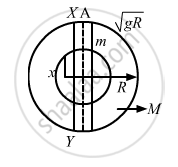Advertisement Remove all ads

# P Assume that a Tunnel is Dug Across the Earth (Radius = R) Passing Through Its Centre. Find the Time a Particle Takes to Cover the Length of the Tunnel If - Physics

Sum

Assume that a tunnel is dug across the earth (radius = R) passing through its centre. Find the time a particle takes to cover the length of the tunnel if (a) it is projected into the tunnel with a speed of $\sqrt{gR}$ (b) it is released from a height R above the tunnel (c) it is thrown vertically upward along the length of tunnel with a speed of $\sqrt{gR}$

Advertisement Remove all ads

#### SolutionGiven:
Radius of the earth is R.
Let be the total mass of the earth and $\rho$ be the density.
Let mass of the part of earth having radius x be M.

$\therefore \frac{M'}{M} = \frac{\rho \times \frac{4}{3}\pi x^3}{\rho \times \frac{4}{3}\pi R^3} = \frac{x^3}{R^3}$

$\Rightarrow M' = \frac{M x^3}{R^3}$

Force on the particle is calculated as,

$F_x = \frac{GM'm}{x^2}$

$= \frac{GMm}{R^3}x \ldots\left( 1 \right)$

Now, acceleration $\left( a_x \right)$ of mass M' at that position is given by,

$a_x = \frac{GM}{R^3}x$

$\Rightarrow \frac{a_x}{x} = \omega^2 = \frac{GM}{R^3} = \frac{g}{R} \left( \because g = \frac{GM}{R^2} \right)$

$\text{So, Time period of oscillation ,} T = 2\pi\sqrt{\left( \frac{R}{g} \right)}$

(a) Velocity-displacement equation in S.H.M is written as,

$V = \omega\sqrt{\left( A^2 - y^2 \right)}$

where,   A  is  the  amplitude;   and                              y  is  the  displacement .

When the particle is at y = R,
The velocity of the particle is $\sqrt{gR}$ and $\omega = \sqrt{\frac{g}{R}}$

On substituting these values in the velocity-displacement equation, we get:

$\sqrt{gR} = \sqrt{\frac{g}{R}}\sqrt{A^2 - R^2}$

$\Rightarrow R^2 = A^2 - R^2$

$\Rightarrow A = \sqrt{2R}$

Let t1 and t2 be the time taken by the particle to reach the positions X and Y.
Now, phase of the particle at point X will be greater than $\frac{\pi}{2}$ but less than $\pi$

Also, the phase of the particle on reaching Y will be greater than $\pi$ but less than $\frac{3\pi}{2}$

Displacement-time relation is given by,
y = A sin ωt

Substituting y = R and A =$\sqrt{2R}$ , in the above relation , we get :

$R = \sqrt{2}R \sin \omega t_1$

$\Rightarrow \omega t_1 = \frac{3\pi}{4}$

Also,

$R = \sqrt{2}R \sin \omega t_2$

$\Rightarrow \omega t_2 = \frac{5\pi}{4}$

$\text{So}, \omega\left( t_2 - t_1 \right) = \frac{\pi}{2}$

$\Rightarrow t_2 - t_1 = \frac{\pi}{2\omega} = \frac{\pi}{2\left( \sqrt{\frac{g}{R}} \right)}$

Time taken by the particle to travel from X to Y:

$t_2 - t_1 = \frac{\pi}{2\omega} = \frac{\pi}{2}\sqrt{\frac{R}{g}}$ s
(b) When the body is dropped from a height R

Using the principle of conservation of energy, we get:
Change in P.E. = Gain in K.E.

$\Rightarrow \frac{GMm}{R} - \frac{GMm}{2R} = \frac{1}{2}m v^2$

$\Rightarrow v = \sqrt{\left( gR \right)}$

As the velocity is same as that at X, the body will take the same time to travel XY.

(c) The body is projected vertically upwards from the point X with a velocity $\sqrt{gR}$.Its velocity becomes zero as it reaches the highest point.
The velocity of the body as it reaches X again will be,

$v = \sqrt{\left( gR \right)}$

Hence, the body will take same time i.e.

$\frac{\pi}{2}\sqrt{\left( \frac{R}{g} \right)}$s to travel XY.
Is there an error in this question or solution?
Advertisement Remove all ads

#### APPEARS IN

HC Verma Class 11, 12 Concepts of Physics 1
Chapter 12 Simple Harmonics Motion
Q 41 | Page 255
Advertisement Remove all ads

#### Video TutorialsVIEW ALL 

Advertisement Remove all ads
Share
Notifications

View all notifications

Forgot password?